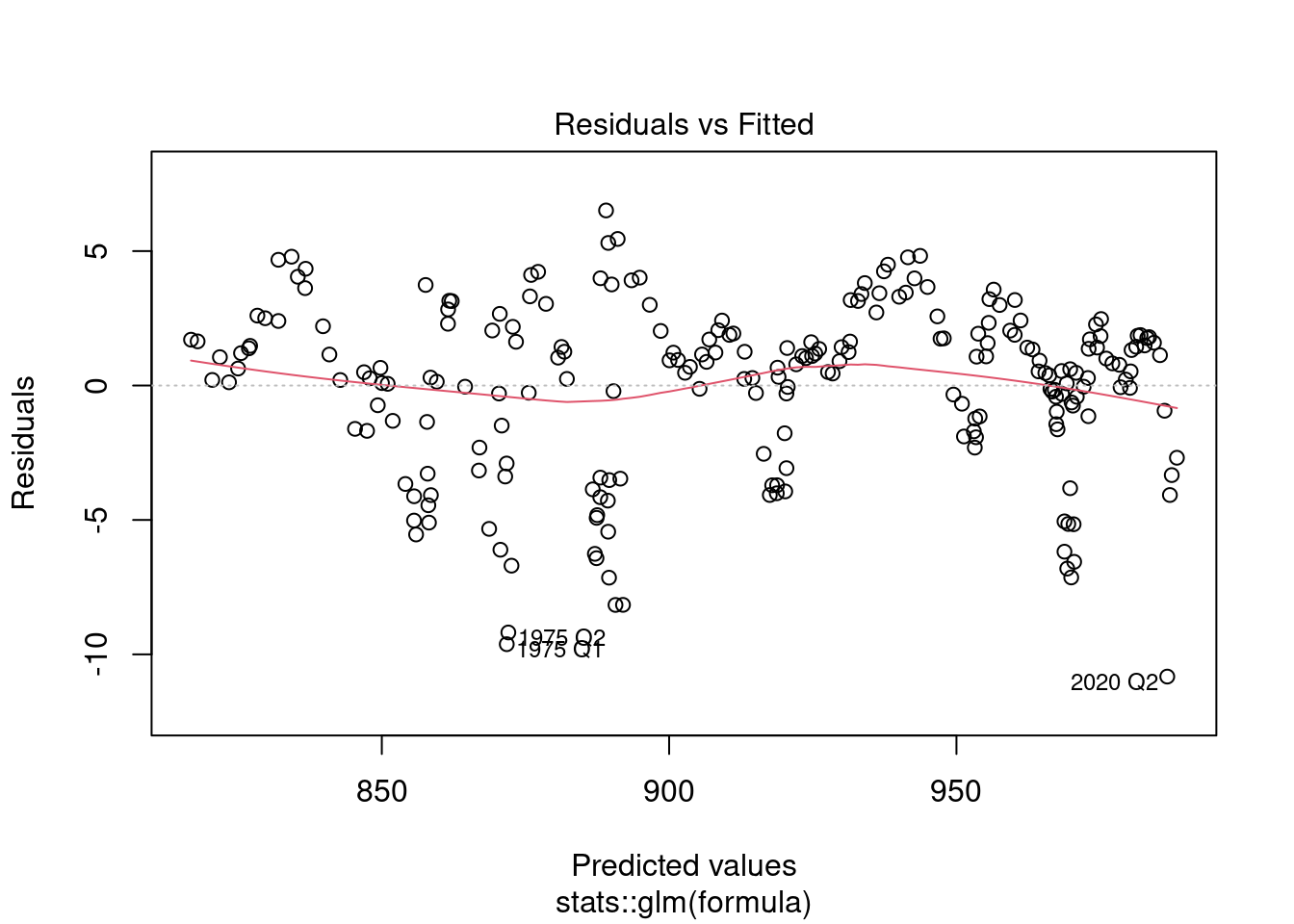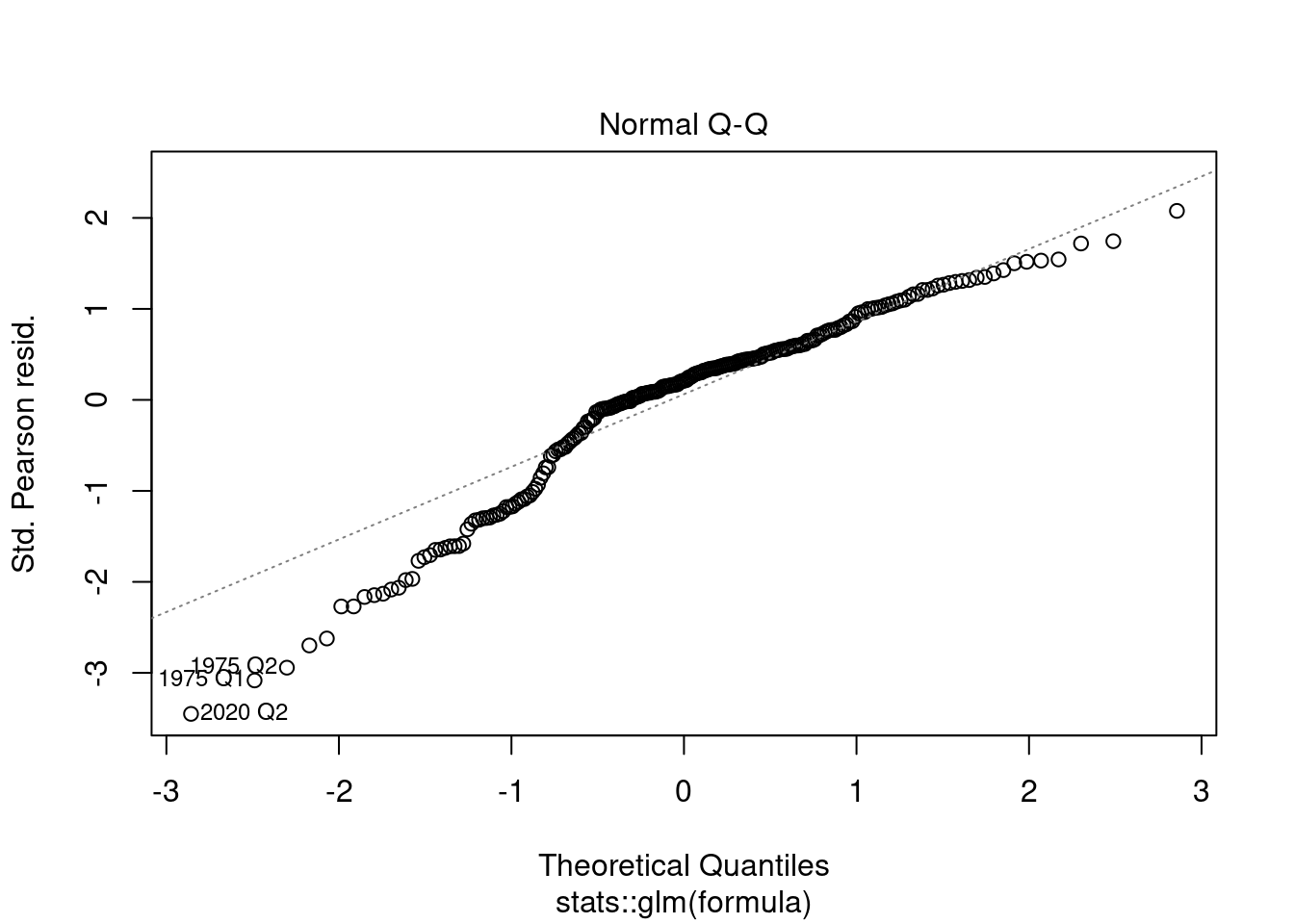# Introduction

The `neverhpfilter` package consists of 2 `functions`, 12 economic data sets, Robert Shiller’s U.S. Stock Market and CAPE Ratio data from 1871 through 2019, and a `data.frame` containing the original filter estimates found on table 2 of Hamilton (2017) <doi:10.3386/w23429>. All data objects are stored as `.Rdata` files in eXtensible Time Series (`xts`) format.

One of the first things to know about the `neverhpfilter` package is that it’s functions accept and output, `xts` objects.

An `xts` object is a `list` consisting of a `vector` index of some date/time class paired with a `matrix` object containing data of type `numeric`. `data.table` is also heavily used in finance and has efficient date/time indexing capabilities as well. It is useful when working with large data.frame like lists containing vectors of multiple data types of equal length. If using `data.table` or some other index based time series data object, merging the `xts` objects created by functions of this package should be fairly easy. Note `xts` is a dependency listed under the “Suggests” field of `data.table` DESCRIPTION file.

For more information on `xts` objects, go here and here.

## yth_glm

The `yth_glm` function wraps `glm` and primarily exists to model the output for the `yth_filter`. On that note, the function API allows one to use the `...` to pass any additional arguments to `glm`.

The `yth_filter` returns an object of class `glm`, so one can use all generic methods associated with `glm` objects. Here is an example of passing the results of a `yth_glm` model to the `plot` function, which outputs the standard plot diagnostics associated with the method.

``````library(neverhpfilter)

data(GDPC1)

log_RGDP <- 100*log(GDPC1)

gdp_model <- yth_glm(log_RGDP["1960/"], h = 8, p = 4)

plot(gdp_model)``````